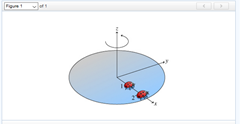# Problem: Two ladybugs sit on a rotating disk, as shown in the figure (the ladybugs are at rest with respect to the surface of the disk and do not slip). (Figure 1) Ladybug 1 is halfway between ladybug 2 and the axis of rotation.Part AWhat is the angular speed of ladybug 1?a. one-half the angular speed of ladybug 2b. the same as the angular speed of ladybug 2c. twice the angular speed of ladybug 2d. one-quarter the angular speed of ladybug 2Part BWhat is the direction of the vector representing the angular velocity of ladybug 2? See the figure for the directions of the coordinate axes.a. +xb. -xc. +yd.-ye. +zf. -z

###### FREE Expert Solution

Part A

Angular velocity:
$\overline{){\mathbf{\omega }}{\mathbf{=}}\frac{\mathbf{∆}\mathbf{\theta }}{\mathbf{∆}\mathbf{t}}}$

Δθ and Δt are equal for both ladybugs.

90% (307 ratings)###### Problem Details

Two ladybugs sit on a rotating disk, as shown in the figure (the ladybugs are at rest with respect to the surface of the disk and do not slip). (Figure 1) Ladybug 1 is halfway between ladybug 2 and the axis of rotation.Part A

What is the angular speed of ladybug 1?

a. one-half the angular speed of ladybug 2

b. the same as the angular speed of ladybug 2

c. twice the angular speed of ladybug 2

d. one-quarter the angular speed of ladybug 2

Part B

What is the direction of the vector representing the angular velocity of ladybug 2? See the figure for the directions of the coordinate axes.

a. +x

b. -x

c. +y

d.-y

e. +z

f. -z